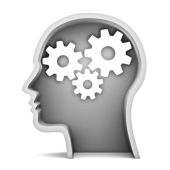LANGUAGE:

# Algebra Puzzles

## Alphametics and CryptarithmsAlphametic puzzles (also called cryptarithms) are arithmetic problems which involve words where each letter stands for unique digit that makes the arithmetic equation true. For instance, this is one of the famous equations. Can you solve it?

``` SEND
MORE
-----
MONEY```

Another example of alphametic puzzles (cryptarithms) goes as follows:

`ALFA + BETA + GAMA = DELTA`

In the following algebra puzzles, replace the same characters by the same numerals so that the mathematical operations are correct.

```ABCB - DEFC = GAFB
:      +      -
DH x   AB =  IEI
-------------------
GGE + DEBB = DHDG```

The same rules apply in this algebra puzzle as well - each letter stands for a unique digit.

```IFIB - EBG = CEH
-     -     +
CCE /  GD =  FE
-----------------
EFF + EED = CBA```

Can you find digits that make equations true in the following alphametic puzzles (cryptarithms)?

```RE + MI = FA
DO + SI = MI
LA + SI = SOL```
`SEVEN + SEVEN + SIX = TWENTY`

hint: Z = 4

``` MOST
MOST
-----
TORZO```
```  SINUS
SINUS
KOSINUS
-------
TANGENS```
```KAJAK
KAJAK
KAJAK
KAJAK
KAJAK
KAJAK
-----
VESLO```
```DVA * DVA = STYRI
D + V + A + D + V + A = S + T + Y + R + I ```
`(AA)B = ABBA`
`ABC + DEF = GHIJ`
`ABC x DEF = 123 456, if A = 1`
`ABCD*D = DCBA`
`ABCD*E = DCBA`
`ABCDEF*3 = BCDEFA`

There are many more algebra puzzles below. Note that answer is always visible once you click the "Display Answer" button. Happy puzzling!

`THC = (T + H + C) x T x H x C`
`AL = LEBKA`
`KOV x KOV = DEDKOV`

Let me conclude with the following easy algebra puzzle. Or is it hard?

`(J+O+I+N+T)3 = JOINT`